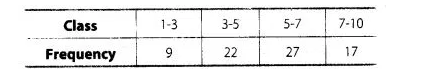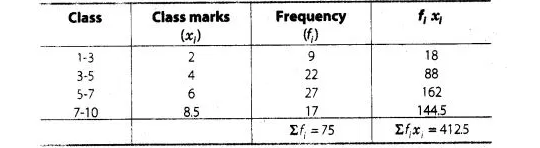# Find the mean of the distribution

Question:

Find the mean of the distributionSolution:

We first, find the class mark xi, of each class and then proceed as follows.Therefore, $\operatorname{mean}(\bar{x})=\frac{\Sigma f_{j} x_{i}}{\Sigma f_{i}}=\frac{412.5}{75}=5.5$

Hence, mean of the given distribution is 5.5.